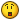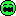# How To...

5 replies to this topic

### #1tonyp

tonyp

Casio Fan

•• Members
•• 34 posts

• Calculators:
TI89, TI89 Titanium, TI-nspire CAS, Casio ClassPad300, Casio fx-6300g, Casio GFX9850GB, Casio 82ES, more

Posted 18 April 2005 - 06:34 PM

Is there an equivalent to the TI-89 exact() function?

DESC: exact() with a single parameter returns the exact (!) number of what's inside the parentheses. Sort of being the opposite of approx(). For example, exact(.3) gives 3/10. You can also use exact(number,precision) to get an estimate to the required precision. For example, exact(3.1415926,.001) returns 22/7 which is an approximation of pi to the third decimal digit.

Anything like this on the Classpad?

tonyp [at] acm [dot] org

Edited by betoe, 19 April 2005 - 08:16 PM.

### #2Daruosh

Daruosh

Casio Freak

•• Members
•• 285 posts
• Gender:Male
• Location:Tehran - Iran
• Interests:Computer Programming, Electronics, Image Processing, Neural Networks, AI, System Development, Calc, Guitar, Music,

• Calculators:
CP 300 OS 3.03, Algebra FX 2.0 Plus ROM 1.05, CG-20

Posted 19 April 2005 - 06:18 PM

use toFrac function

### #3Guest_Guest_*

Guest_Guest_*
•• Guests

Posted 21 April 2005 - 03:49 PM

use toFrac function

<{POST_SNAPBACK}>

Thanks for your response but it's not what I'm looking for. TI's exact(number,precision) gets you the simplest fraction that will get you the closest approximation to the desired precision. toFrac(), on the other hand, simply converts to a fraction which is no different than entering a fractional number and pressing EXE. Using exact() with pi and a relatively good precision, you can get 355/113 which is 3.14159292 which is a very close approximation of pi. Going from the fractional value of pi, it's impossible to get back the 355/113 fraction because it is not equal to pi at any precision.

### #4Lianna

Lianna

Newbie

•• Members
•• 14 posts

• Calculators:
CFX-9850G

Posted 22 May 2005 - 10:19 PM

Is there an equivalent to the TI-89 exact() function?
DESC: exact() with a single parameter returns the exact (!) number of what's inside the parentheses.  Sort of being the opposite of approx().  For example, exact(.3) gives 3/10.  You can also use exact(number,precision) to get an estimate to the required precision.  For example, exact(3.1415926,.001) returns 22/7 which is an approximation of pi to the third decimal digit.
Anything like this on the Classpad?
tonyp [at] acm [dot] org

Well, I don't think so, but:
1) input .3 alone and you should see 3/10 in algebra mode.
2) Define exact1(x,y,z)=propFrac((10^-y)*int(x*10^y)+(10^(z-y))*frac(x*10^y)/(10^z-1))
Use: exact1(decimal, number of set decimal digits, number of digits to repeat)
To convert 1.0(285714) (<- this means 6 repeated digits, 1.0285714285714...):
exact1(1.0285714, 1, 6)
To convert 0.(9) = 0.999999...:
exact1(0.9, 0, 1)

Best regards,
Lianna

### #5Lianna

Lianna

Newbie

•• Members
•• 14 posts

• Calculators:
CFX-9850G

Posted 23 May 2005 - 12:00 AM

3) Brute force (best match) approach, use: exact2(value,precision)
Define exact2(v,p)=exact2b(v,int(1/(4*p)),int(1/(2*p)))
Define exact2b(v,a,b)=exact2c(max(seq(intg(v*x)/x,x,a,b,1)),min(seq(-intg(-v*x)/x,x,a,b,1)),v)
Define exact2c(a,b,c)=piecewise(c-a<b-c,a,b)

Note:
The above is fast enough (15s) only for p>=0.01. Things would be MUCH faster even in Basic (CFX version I wrote some time ago is faster even though it displays partial matches). The problem is, Basic program on Classpad would be less useful, because you can't run Basic in Main. Of course, hardcoded function would be even better.

Pi is the only interesting number I know that has SO good precision at 355/113. Most of the non-fractional real numbers do not have fractional approximations that would be significantly (orders) better than decimal fraction up to the needed precision (compared to denominator)... This could be probably proven... anyone? So usefulness of exact(val,prec) function is questionable.

Just my two cents...
Best regards,
Lianna

### #6Future owner maybe

Future owner maybe

Newbie

•• Members
•• 4 posts

• Calculators:
CASIO FX 82 MS (lost)
HP 49 G+ (broken)
Algebra FX 2.0 PLUS (good!)

Posted 15 February 2006 - 04:28 AM

how to give the polynomial expression inputting the zeros (roots) of the poly?A little stupid question nowadaysThanks!

#### 0 user(s) are reading this topic

0 members, 0 guests, 0 anonymous users